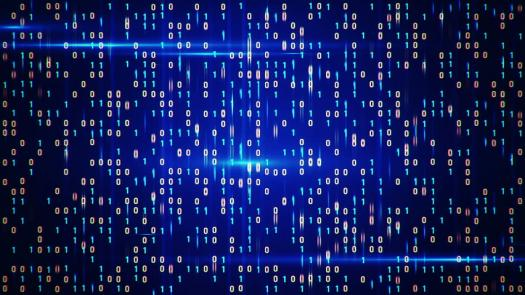# Digital Electronics Internal Assessment 13.3

15 Questions | Attempts: 95
ShareSettings• 1.
A Master-Slave J-K Flip-flop does not suffer from?
• A.

Negative-edge triggering problems

• B.

Positive-edge triggering problems

• C.

Reset problems

• D.

Race hazards

• 2.
A bistable has?
• A.

A single trigger input

• B.

A single active device

• C.

A single output

• D.

Two stable states

• 3.
If a clocked S-R flip flop with its Q output at logic 1 now has both its SET and RESET inputs taken to Logic 1 then after the next clock pulse the Q output will be?
• A.

Logic 1

• B.

Logic 0

• C.

Indeterminate

• D.

Logic 0 momentarily

• 4.
A J-K flip-flop with its Q output at logic 1 has its J input at logic 1 and K input taken to logic 0. After the next clock pulse the Q output will be?
• A.

Indeterminate

• B.

Logic 1

• C.

Logic 0

• D.

Logic 0 momentarily

• 5.
If a J-K flip-flop with its Q output at logic 0 now has its J input taken from logic 0 to logic 1 with its K input remaining at logic 0 then after the next clock pulse the Q output will be?
• A.

Logic 0

• B.

Logic 1

• C.

Indeterminate

• D.

Logic 1 momentarily

• 6.
A Master-Slave J-K flip flop with its Q output at logic 1 now has its J input taken from logic 1 to logic 0 with its K input remaining at logic 1 then after the next clock pulse the Q output will be?
• A.

Logic 1

• B.

Logic 0

• C.

Indeterminate

• D.

Logic 0 momentarily

• 7.
In an asynchronous Counter the flip-flops change state
• A.

Simultaneously

• B.

In an orderly sequence

• C.

After each clock pulse

• D.

Before each clock pulse

• 8.
A Schmitt Trigger buffer gate would perform the following function?
• A.

Converts a Sine to Square wave

• B.

Changes an AC signal to DC

• C.

Changes an DC signal to AC

• D.

Latches on once an input is received

• 9.
The circuit below represents which sequential logic circuit?
• A.

SR Latch

• B.

JK flip flop

• C.

D type latch

• D.

T Type Latch

• 10.
The circuit below represents the function of which sequential logic circuit?
• A.

SR Latch

• B.

JK flip flop

• C.

D type latch

• D.

T Type Latch

• 11.
The following symbol represents a?
• A.

3 Input buffer

• B.

SR Latch

• C.

Tri state device

• D.

Clock generator

• 12.
A 5-bit binary counter set at 00000 will reset on clock pulse number?
• A.

28

• B.

16

• C.

48

• D.

32

• 13.
In a synchronous counter the flip-flops ALWAYS change state?
• A.

Independently of the clock pulse input

• B.

Simultaneously

• C.

After each clock pulse

• D.

Before each clock pulse

• 14.
Tri-state logic gates are characterised by having?
• A.

A control pin to set the output to a high impedance

• B.

A control pin to set the output to a low impedance

• C.

A control pin to set the output to logic 1

• D.

A control pin to set the output to logic 0

• 15.
A Divide-by-five Counter can be made from?
• A.

Two bistables and two NAND gates

• B.

Three bistables and one NAND gate

• C.

Four bistables and one NAND gate

• D.

Five bistables

## Related TopicsBack to top
×

Wait!
Here's an interesting quiz for you.